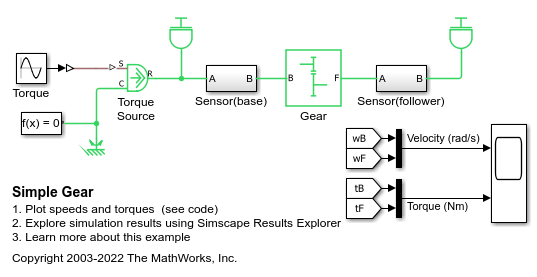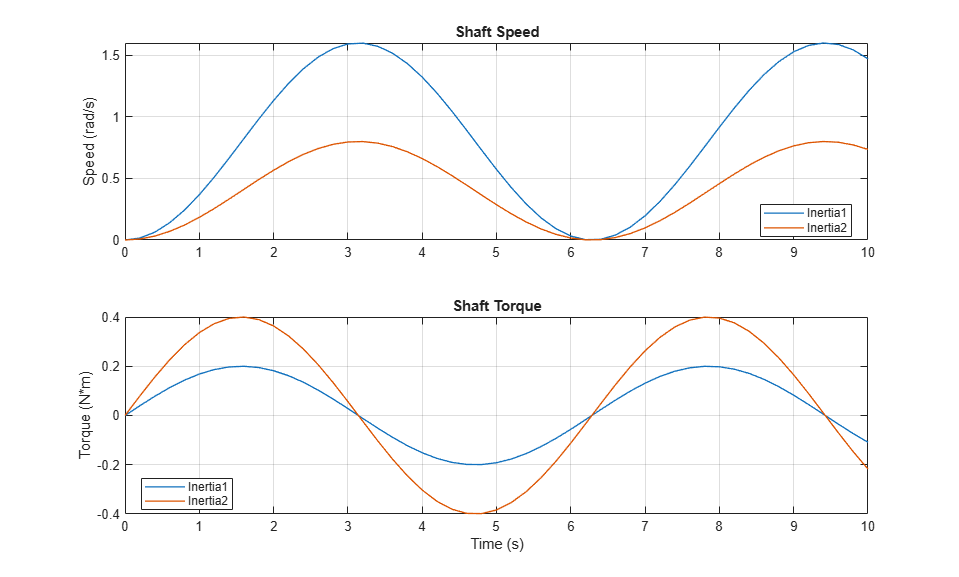# Simple Gear

This example shows a simple gear coupling two inertias (shafts). The gear ratio between the follower (F) and base (B) is 2:1. Thus the angular velocity of the follower shaft is half the angular velocity of the base shaft. The follower shaft torque is twice the base shaft torque.

An alternative to adding sensors to your model is to use Simscape™ data logging. This example has data logging enabled, passing results to the workspace variable 'simlog_SimpleGear'. For example, entering 'plot(simlog_SimpleGear.Gear.B.w)' at the command line plots the angular velocity at port B of the Gear block. See the Data Logging Example in the Simscape User Guide for further details.

### Model### Simulation Results from Simscape Logging

The plots below show the speeds and torques applied to two shafts connected by a gearbox.